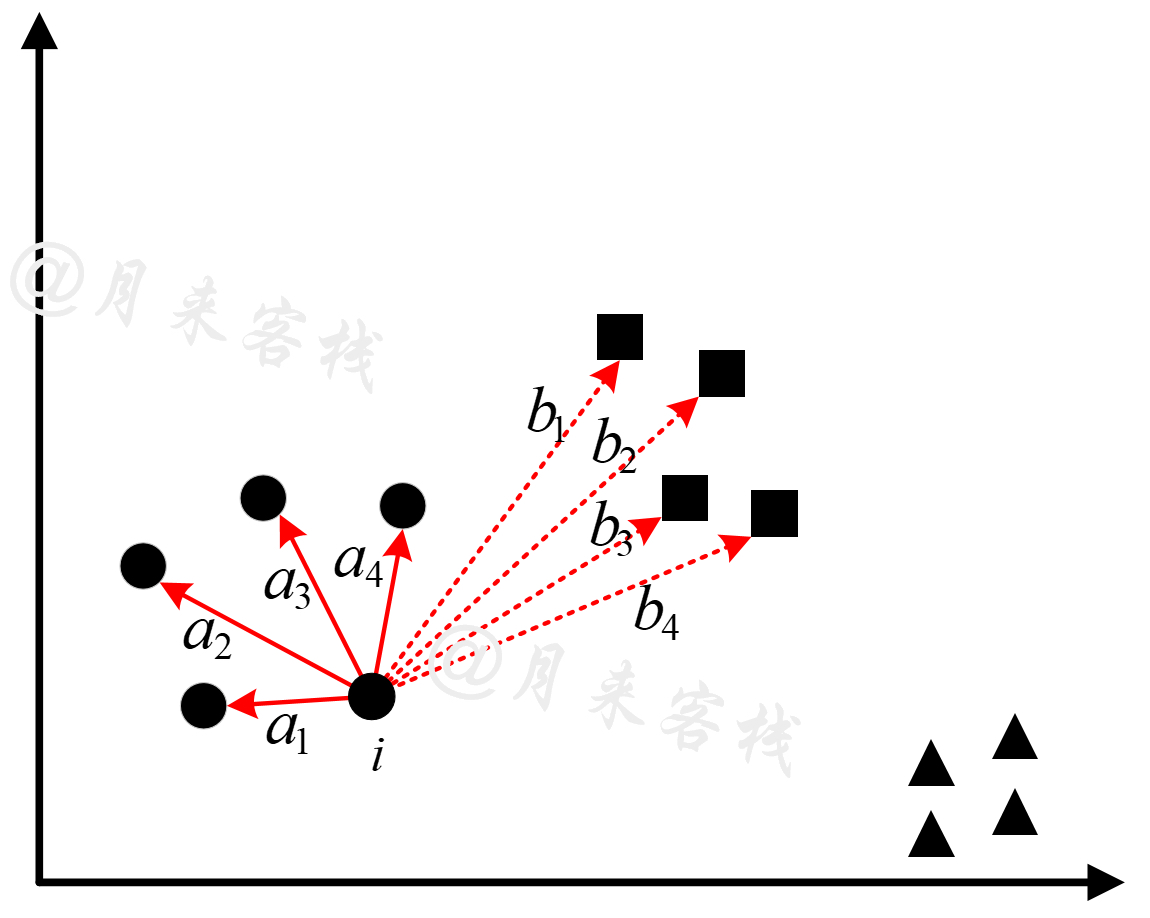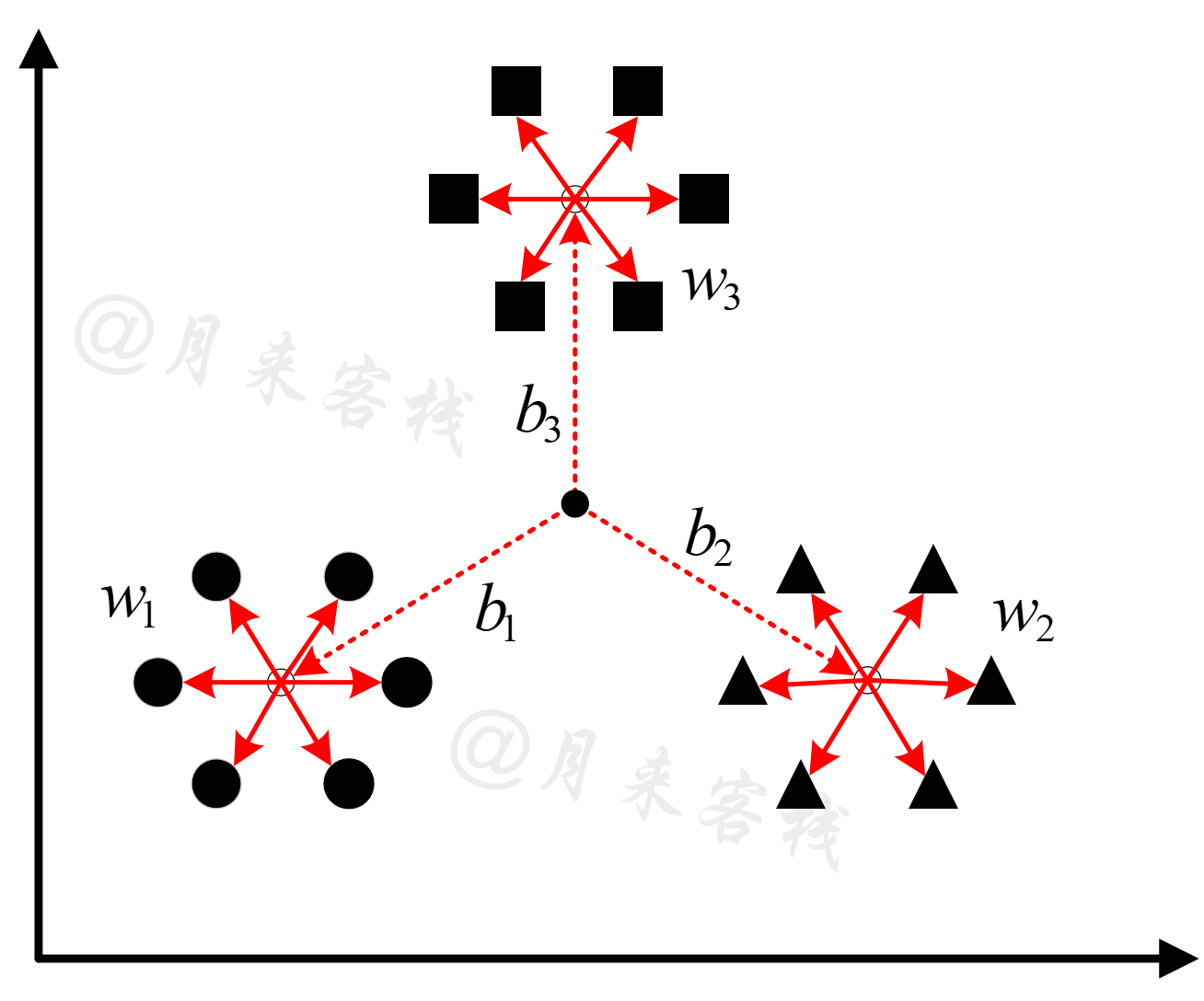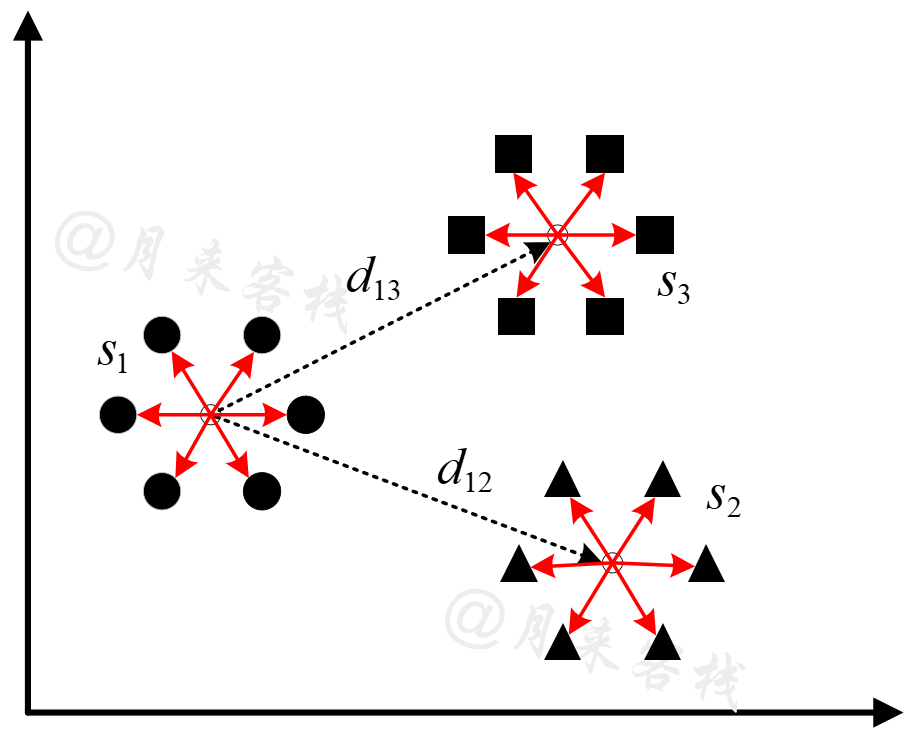### 2 Silhouette Coefficient Index

#### 2.1 原理$\begin{array}{rl}a\left(i\right)& =\frac{{a}_{1}+{a}_{2}+{a}_{3}+{a}_{4}}{4}\\ b\left(i\right)& =\frac{{b}_{1}+{b}_{2}+{b}_{3}+{b}_{4}}{4}\end{array}\phantom{\rule{0.278em}{0ex}}\phantom{\rule{0.278em}{0ex}}\phantom{\rule{0.278em}{0ex}}\phantom{\rule{0.278em}{0ex}}\phantom{\rule{0.278em}{0ex}}\phantom{\rule{0.278em}{0ex}}\phantom{\rule{0.278em}{0ex}}\phantom{\rule{0.278em}{0ex}}\left(1\right)$

$s\left(i\right)=\frac{b\left(i\right)-a\left(i\right)}{max\left\{a\left(i\right),b\left(i\right)\right\}}\phantom{\rule{0.278em}{0ex}}\phantom{\rule{0.278em}{0ex}}\phantom{\rule{0.278em}{0ex}}\phantom{\rule{0.278em}{0ex}}\phantom{\rule{0.278em}{0ex}}\phantom{\rule{0.278em}{0ex}}\phantom{\rule{0.278em}{0ex}}\phantom{\rule{0.278em}{0ex}}\left(3\right)$

$s=\frac{1}{n}\sum _{i=1}^{n}s\left(i\right)\phantom{\rule{0.278em}{0ex}}\phantom{\rule{0.278em}{0ex}}\phantom{\rule{0.278em}{0ex}}\phantom{\rule{0.278em}{0ex}}\phantom{\rule{0.278em}{0ex}}\phantom{\rule{0.278em}{0ex}}\phantom{\rule{0.278em}{0ex}}\phantom{\rule{0.278em}{0ex}}\left(4\right)$

### 2.2 实现

xxxxxxxxxx241def get_silhouette_coefficient(X, labels):2    n_clusters = np.unique(labels).shape3    s = []4    for k in range(n_clusters):  # 遍历每一个簇5        index = (labels == k)  # 取对应簇所有样本的索引6        x_in_cluster = X[index]  # 去对应簇中的所有样本7        for sample in x_in_cluster:  # 计算每个样本的轮廓系数8            a = ((sample - x_in_cluster) ** 2).sum(axis=1)9            a = np.sqrt(a).sum() / (len(a) - 1)  # 去掉当前样本点与当前样本点的组合计数10            nearest_cluster_id = None11            min_dist2 = np.inf12            for c in range(n_clusters):  # 寻找距离当前样本点最近的簇13                if k == c:14                    continue15                centroid = X[labels == c].mean(axis=0)16                dist2 = ((sample - centroid) ** 2).sum()17                if dist2 < min_dist2:18                    nearest_cluster_id = c19                    min_dist2 = dist220            x_nearest_cluster = X[labels == nearest_cluster_id]21            b = ((sample - x_nearest_cluster) ** 2).sum(axis=1)22            b = np.sqrt(b).mean()23            s.append((b - a) / np.max([a, b]))24    return np.mean(s)

xxxxxxxxxx161import numpy as np2from sklearn.cluster import KMeans3from sklearn.datasets import load_iris4from sklearn.metrics import silhouette_score5
6def test_silhouette_score():7    x, y = load_iris(return_X_y=True)8    model = KMeans(n_clusters=3)9    model.fit(x)10    y_pred = model.predict(x)11    print(f"轮廓系数 by sklearn: {silhouette_score(x, y_pred)}")12    print(f"轮廓系数 by ours: {get_silhouette_coefficient(x, y_pred)}")13
14
15if __name__ == '__main__':16    test_silhouette_score()

xxxxxxxxxx21轮廓系数 by sklearn: 0.55281901235640912轮廓系数 by ours: 0.5528190123564101

### 3 Calinski-Harabasz Index

#### 3.1 原理$\begin{array}{rl}W& =\sum _{k=1}^{K}{w}_{k}=\sum _{k=1}^{K}\sum _{x\in {C}_{k}}\left(x-{c}_{k}{\right)}^{2}\\ B& =\sum _{k=1}^{K}{b}_{k}=\sum _{k=1}^{K}{n}_{k}\left({c}_{k}-c{\right)}^{2}\\ s& =\frac{B}{K-1}/\frac{W}{n-K}=\frac{B}{W}\cdot \frac{n-K}{K-1}\end{array}\phantom{\rule{0.278em}{0ex}}\phantom{\rule{0.278em}{0ex}}\phantom{\rule{0.278em}{0ex}}\phantom{\rule{0.278em}{0ex}}\phantom{\rule{0.278em}{0ex}}\phantom{\rule{0.278em}{0ex}}\phantom{\rule{0.278em}{0ex}}\phantom{\rule{0.278em}{0ex}}\left(5\right)$

#### 3.2 实现

xxxxxxxxxx151def get_calinski_harabasz(X, labels):2    n_samples = X.shape3    n_clusters = np.unique(labels).shape4    betw_disp = 0.  # 所有的簇间距离和5    within_disp = 0.  # 所有的簇内距离和6    global_centroid = np.mean(X, axis=0)  # 全局簇中心7    for k in range(n_clusters):  # 遍历每一个簇8        x_in_cluster = X[labels == k]  # 取当前簇中的所有样本9        centroid = np.mean(x_in_cluster, axis=0)  # 计算当前簇的簇中心10        within_disp += np.sum((x_in_cluster - centroid) ** 2)11        betw_disp += len(x_in_cluster) * np.sum((centroid - global_centroid) ** 2)12
13    return (1. if within_disp == 0. else14            betw_disp * (n_samples - n_clusters) /15            (within_disp * (n_clusters - 1.)))

xxxxxxxxxx101def test_calinski_harabasz_score():2    x, y = load_iris(return_X_y=True)3    model = KMeans(n_clusters=3)4    model.fit(x)5    y_pred = model.predict(x)6    print(f"方差比 by sklearn: {calinski_harabasz_score(x, y_pred)}")7    print(f"方差比 by ours: {get_calinski_harabasz(x, y_pred)}")8
9if __name__ == '__main__':10    test_calinski_harabasz_score()

xxxxxxxxxx21方差比 by sklearn: 561.627756629622方差比 by ours: 561.62775662962

### 4 Davies-Bouldin Index

#### 4.1 原理${R}_{ij}=\frac{{s}_{i}+{s}_{j}}{{d}_{ij}}\phantom{\rule{0.278em}{0ex}}\phantom{\rule{0.278em}{0ex}}\phantom{\rule{0.278em}{0ex}}\phantom{\rule{0.278em}{0ex}}\phantom{\rule{0.278em}{0ex}}\phantom{\rule{0.278em}{0ex}}\phantom{\rule{0.278em}{0ex}}\phantom{\rule{0.278em}{0ex}}\left(6\right)$

$DB=\frac{1}{K}\sum _{i,j=1}^{K}\underset{i\ne j}{max}{R}_{ij}\phantom{\rule{0.278em}{0ex}}\phantom{\rule{0.278em}{0ex}}\phantom{\rule{0.278em}{0ex}}\phantom{\rule{0.278em}{0ex}}\phantom{\rule{0.278em}{0ex}}\phantom{\rule{0.278em}{0ex}}\phantom{\rule{0.278em}{0ex}}\phantom{\rule{0.278em}{0ex}}\left(7\right)$

#### 4.2 实现

xxxxxxxxxx131def get_davies_bouldin(X, labels):2    n_clusters = np.unique(labels).shape3    centroids = np.zeros((n_clusters, len(X)), dtype=float)4    s_i = np.zeros(n_clusters)5    for k in range(n_clusters):  # 遍历每一个簇6        x_in_cluster = X[labels == k]  # 取当前簇中的所有样本7        centroids[k] = np.mean(x_in_cluster, axis=0)  # 计算当前簇的簇中心8        s_i[k] = pairwise_distances(x_in_cluster, [centroids[k]]).mean()  #9    centroid_distances = pairwise_distances(centroids)  # [K,K]10    combined_s_i_j = s_i[:, None] + s_i  # [K,k]11    centroid_distances[centroid_distances == 0] = np.inf12    scores = np.max(combined_s_i_j / centroid_distances, axis=1)13    return np.mean(scores)

xxxxxxxxxx61centroid_distances = [[0.         3.35693455 5.01756852]2                      [3.35693455 0.         1.7971818 ]3                      [5.01756852 1.7971818  0.        ]]4combined_s_i_j = [[0.96341047 1.21985761 1.20154379]5                  [1.21985761 1.47630474 1.45799092]6                  [1.20154379 1.45799092 1.4396771 ]]

xxxxxxxxxx101def test_davies_bouldin_score():2    x, y = load_iris(return_X_y=True)3    model = KMeans(n_clusters=3)4    model.fit(x)5    y_pred = model.predict(x)6    print(f"db_score by sklearn: {davies_bouldin_score(x, y_pred)}")7    print(f"db_score by ours: {get_davies_bouldin(x, y_pred)}")8
9if __name__ == '__main__':10    test_davies_bouldin_score()

xxxxxxxxxx21db_score by sklearn: 0.66197154650075112db_score by ours: 0.6619715465007511

### 引用

 Rousseeuw P J. Silhouettes: a graphical aid to the interpretation and validation of cluster analysis[J]. Journal of computational and applied mathematics, 1987, 20: 53-65.

 Caliński T, Harabasz J. A dendrite method for cluster analysis[J]. Communications in Statistics-theory and Methods, 1974, 3(1): 1-27.

 Davies, David L.; Bouldin, Donald W. (1979). “A Cluster Separation Measure” IEEE Transactions on Pattern Analysis and Machine Intelligence. PAMI-1 (2): 224-227.